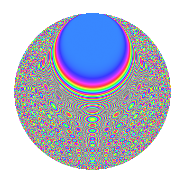# Properties

 Label 402.2.nLevel 402 Weight 2 Character orbit n Rep. character $$\chi_{402}(11,\cdot)$$ Character field $$\Q(\zeta_{66})$$ Dimension 440 Newform subspaces 2 Sturm bound 136 Trace bound 2

# Related objects

## Defining parameters

 Level: $$N$$ $$=$$ $$402 = 2 \cdot 3 \cdot 67$$ Weight: $$k$$ $$=$$ $$2$$ Character orbit: $$[\chi]$$ $$=$$ 402.n (of order $$66$$ and degree $$20$$) Character conductor: $$\operatorname{cond}(\chi)$$ $$=$$ $$201$$ Character field: $$\Q(\zeta_{66})$$ Newform subspaces: $$2$$ Sturm bound: $$136$$ Trace bound: $$2$$ Distinguishing $$T_p$$: $$5$$

## Dimensions

The following table gives the dimensions of various subspaces of $$M_{2}(402, [\chi])$$.

Total New Old
Modular forms 1440 440 1000
Cusp forms 1280 440 840
Eisenstein series 160 0 160

## Trace form

 $$440q + 22q^{4} + q^{6} + 12q^{7} - 2q^{9} + O(q^{10})$$ $$440q + 22q^{4} + q^{6} + 12q^{7} - 2q^{9} - 8q^{12} - 12q^{13} - 8q^{15} + 22q^{16} + 3q^{18} + 50q^{19} + 28q^{21} + 24q^{22} + 20q^{24} - 20q^{25} - 12q^{28} + 6q^{30} - 4q^{31} - 16q^{33} - 6q^{34} + q^{36} + 20q^{37} - 54q^{39} - 44q^{43} + 22q^{45} + 60q^{46} + 19q^{48} - 54q^{49} + 3q^{51} + 44q^{52} + 28q^{54} - 288q^{55} + 3q^{57} - 88q^{58} + 4q^{60} - 296q^{61} + 42q^{63} - 44q^{64} - 48q^{67} - 30q^{69} - 308q^{70} - 334q^{73} - 99q^{75} - 100q^{76} - 48q^{78} + 24q^{79} + 6q^{81} - 76q^{82} + 6q^{84} - 60q^{85} + 48q^{87} - 12q^{88} - 26q^{90} + 8q^{91} + 84q^{93} + 44q^{94} + q^{96} - 138q^{97} - 32q^{99} + O(q^{100})$$

## Decomposition of $$S_{2}^{\mathrm{new}}(402, [\chi])$$ into newform subspaces

Label Dim. $$A$$ Field CM Traces $q$-expansion
$$a_2$$ $$a_3$$ $$a_5$$ $$a_7$$
402.2.n.a $$220$$ $$3.210$$ None $$-11$$ $$1$$ $$0$$ $$6$$
402.2.n.b $$220$$ $$3.210$$ None $$11$$ $$-1$$ $$0$$ $$6$$

## Decomposition of $$S_{2}^{\mathrm{old}}(402, [\chi])$$ into lower level spaces

$$S_{2}^{\mathrm{old}}(402, [\chi]) \cong$$ $$S_{2}^{\mathrm{new}}(201, [\chi])$$$$^{\oplus 2}$$

## Hecke characteristic polynomials

There are no characteristic polynomials of Hecke operators in the database